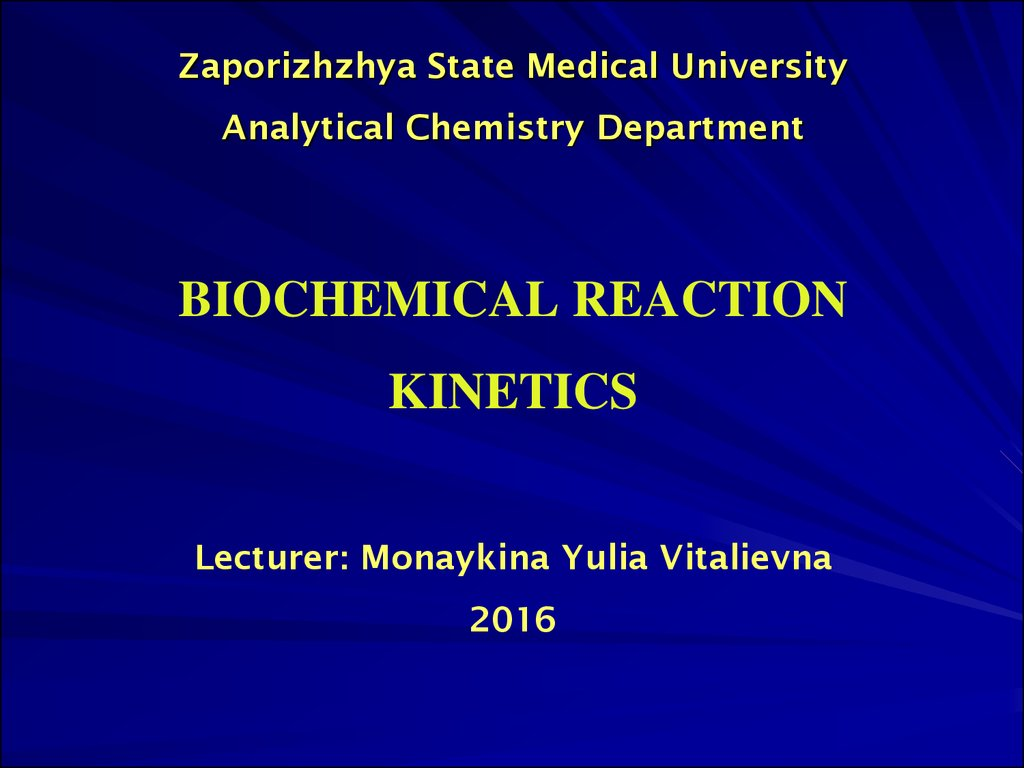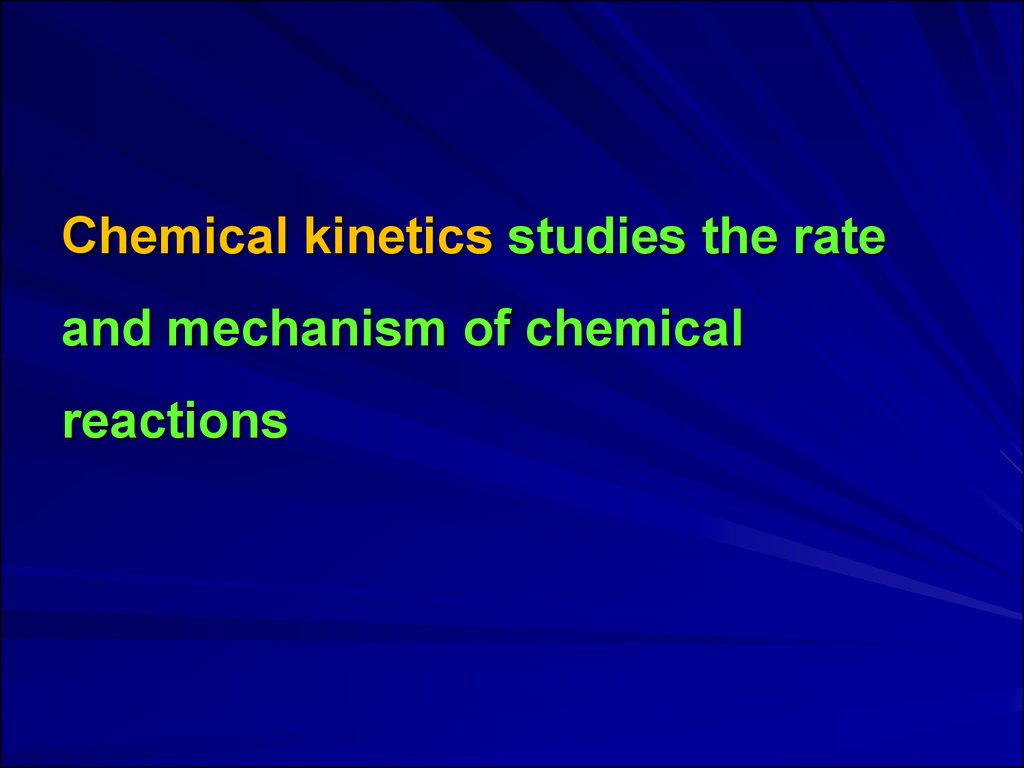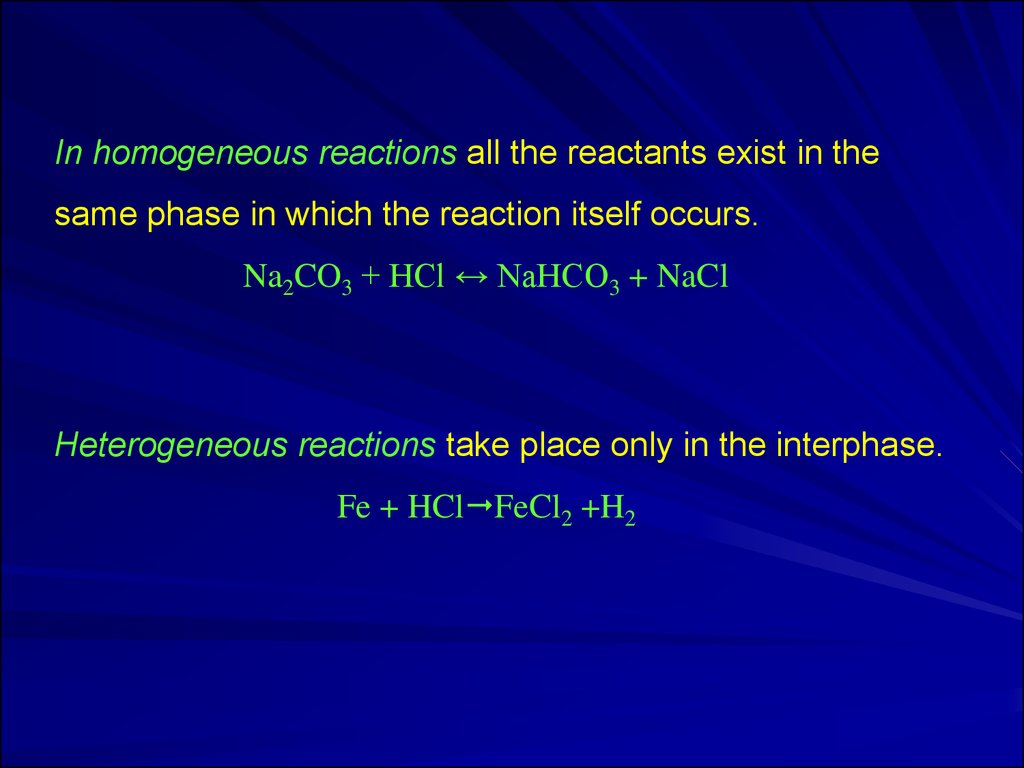# Biochemical reaction kinetics

## 2.

Chemical kinetics studies the rate
and mechanism of chemical
reactions

## 3. In homogeneous reactions all the reactants exist in the same phase in which the reaction itself occurs. Na2CO3 + HCl ↔ NaHCO3 + NaCl Heterogeneous reactions take place only in the interphase. Fe + HClFeCl2 +H2

In homogeneous reactions all the reactants exist in the
same phase in which the reaction itself occurs.
Na2CO3 + HCl ↔ NaHCO3 + NaCl
Heterogeneous reactions take place only in the interphase.
Fe + HCl FeCl2 +H2

## 4.

Single-stage reactions are called simple
(or elementary) reactions.
Multistage reactions include few simple
reactions and are called complex
(or non-elementary) reactions.
All biochemical reactions are non-elementary.

## 5.

The dependence of the reaction rate on the
concentration of reactants is described by the law of
mass action discovered by N.Beketov, C. Guldberg
and P. Waage in 1967:
«At constant temperature the rate of chemical
reaction is in direct proportion to the product of
reactant concentrations in the degree of their
stoichiometric coefficients».

## 6.

Mathematical expression of the law of
mass action is called a
kinetic equation
or
rate law of the reaction.

## 7.

Molecularity of the reaction
is determined by the number of
molecules which interact and take part
in an elementary act of the reaction.

## 8. Arrenius Equation establishes a connection between the reaction rate constant, activation energy and temperature:

k A e
Ea
RT
e
is base of natural logarithm;
R
T
A
is universal gas constant (8.314 J / mol · K);
is absolute temperature, K;
is pre-exponential factor.

## 9.

Catalysis is the change of chemical
reactions rate under the influence of
substances, the amount and nature of
which, after completion of the reaction are
the same as before the reaction.
Catalyst is a substance that influences the
rate of chemical processes without changing
its own chemical composition.

## 10.

Enzymes are catalysts of the chemical
reactions in the body.
An enzyme is a protein that catalyses a
chemical reaction by lowering the activation
energy.